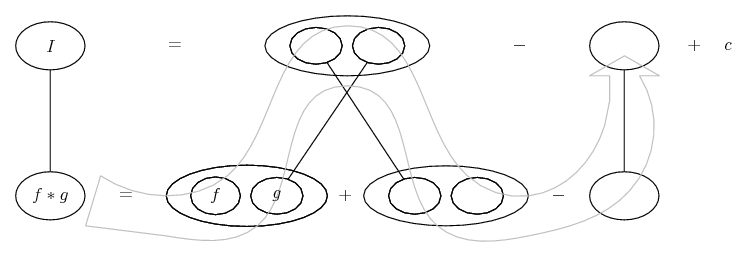# Thread: integration

1. ## integration

I want to integrate sec x(2x +x^2 tanx). The wolfram site does not give the steps.

2. ## Re: integrationOriginally Posted by Stuck ManI want to integrate sec x(2x +x^2 tanx). The wolfram site does not give the steps.
Which is surprising as if you differentiate x^2 sec x you get, directly, the expansion of your integrand.

I.e., expand, and do integration by parts on either part of the sum.

Just in case a picture helps...

Spoiler:... where (key in spoiler) ...

Spoiler:... is the chain rule. Straight continuous lines differentiate downwards (integrate up) with respect to the main variable (in this case x), and the straight dashed line similarly but with respect to the dashed balloon expression (the inner function of the composite which is subject to the chain rule).

But this is wrapped inside the legs-uncrossed version of...... the product rule, where, again, straight continuous lines are differentiating downwards with respect to x.... is lazy integration by parts, doing without u and v.

Though of course you find that you don't need to subtract anything other than the rest of the integrand, i.e. you had the whole of a product-rule derivative in the first place.

__________________________________________________ __________

Don't integrate - balloontegrate!

Balloon Calculus; standard integrals, derivatives and methods

Balloon Calculus Drawing with LaTeX and Asymptote!

3. ## Re: integrationOriginally Posted by Stuck ManI want to integrate sec x(2x +x^2 tanx). The wolfram site does not give the steps.
Let $\displaystyle u = x^2\Rightarrow du = 2x\,dx$ and $\displaystyle v = \sec x\Rightarrow dv = \sec x\tan x\,dx.$ Then

$\displaystyle \int\sec x\left(2x + x^2\tan x\right)\,dx$

$\displaystyle =\int2x\sec x\,dx + \int x^2\sec x\tan x\,dx$

$\displaystyle = \int v\,du + \int u\,dv$

$\displaystyle = \int v\,du + uv - \int v\,du$ (integration by parts)

$\displaystyle = uv + C = x^2\sec x + C.$

Edit: Forgot my constant!

4. ## Re: integration

u = tanx
du = sec²x

∫ u du

(1/2)u² + C

(1/2)tan²x + C

If you use the pythagorean theorem

(1/2)(sec²x - 1) + C

(1/2)sec²x - (1/2) + C

you can combine the two constants

(1/2)sec²x + C

So now you can see that they are both equivalent with the exception of the constant, but since you can choose ANY number as the constant they'll be equivalent anyway.

Geometry Tutor Online
Online Calculus Tutoring

#### Search Tags

integration